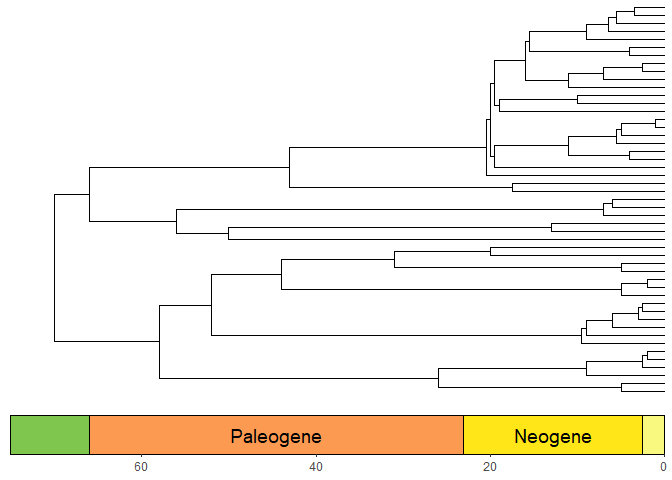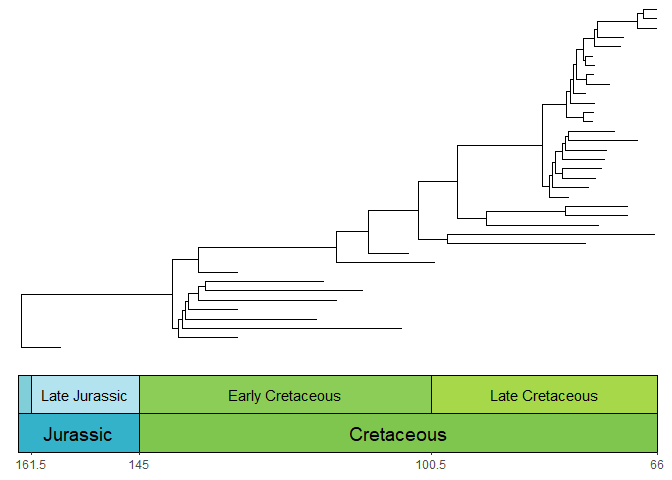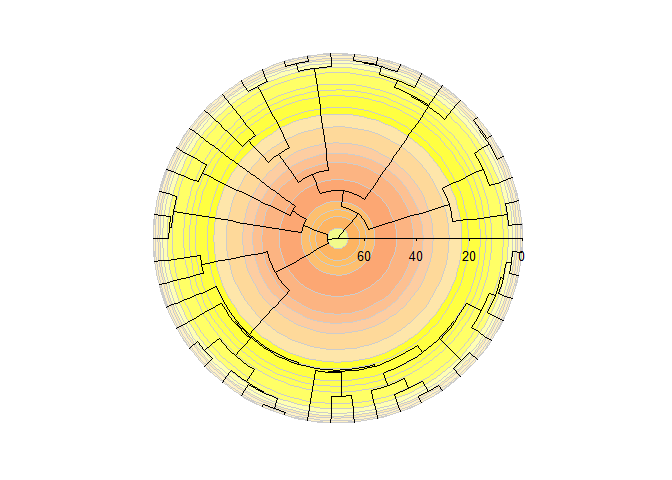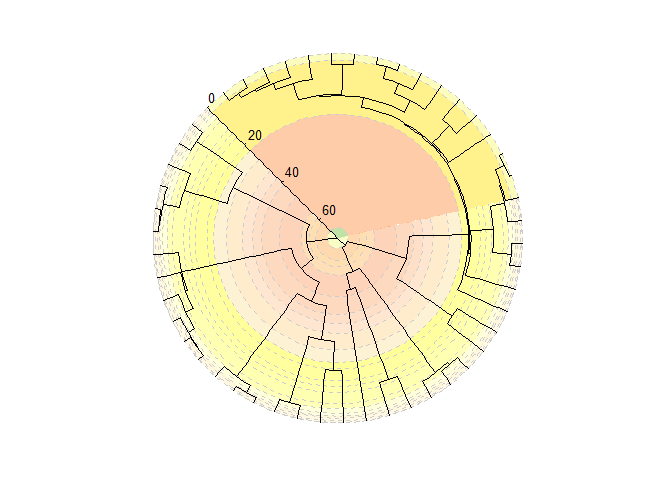# Adding geological timescales to phylogenies

coord_geo() is also designed to work smoothly with phylogenies that are plotted with the ggtree package. First let’s load some important packages.

# Load deeptime
library(deeptime)
library(ggplot2)
library(dplyr)
library(magrittr) # for piping
library(ggtree)
# Load phytools for some example data
library(phytools)
data(mammal.tree)
# Load paleotree for some example fossil data
library(paleotree)
data(RaiaCopesRule)

## Timescales and phylogenies

Now, let’s plot some phylogenies with timescales! Note that by default the time axis increases toward the tips for trees plotted with ggtree(), so you must use the revts() function from ggtree to change the x-axis values to increasing negative values. Note that once you’ve done this, you will need to set neg = TRUE in coord_geo(). Furthermore, any time axis limits that you set will need to be negative. Here we also use the scale_x_continuous() function to relabel the negative x-axis values with positive labels.

p <- ggtree(mammal.tree) +
coord_geo(xlim = c(-75, 0), ylim = c(-2, Ntip(mammal.tree)), neg = TRUE, abbrv = FALSE) +
scale_x_continuous(breaks = seq(-80, 0, 20), labels = abs(seq(-80, 0, 20))) +
theme_tree2()
revts(p)### Phylogenies with only fossil taxa

Many phylogenies only have fossil taxa in them (i.e., non-ultrametric). These can be handled by coord_geo() by using position_nudge() function. In most cases, you will want to nudge the time axis values (in this case, the x-axis) by the root.time of your phylogeny. Also, note that we have modified plot.margin here to accommodate the stacked timescale.

ggtree(ceratopsianTreeRaia, position = position_nudge(x = -ceratopsianTreeRaia$root.time)) + coord_geo( xlim = c(-163.5, -66), ylim = c(-2, Ntip(ceratopsianTreeRaia)), pos = list("bottom", "bottom"), skip = c("Paleocene", "Middle Jurassic"), dat = list("epochs", "periods"), abbrv = FALSE, size = list(4, 5), neg = TRUE, center_end_labels = TRUE ) + scale_x_continuous(breaks = -rev(epochs$max_age), labels = rev(epochs\$max_age)) +
theme_tree2() +
theme(plot.margin = margin(7, 11, 7, 11))## Circular phylogenies

Phylogenies can be plotted in a circular layout with a timescale background using the coord_geo_polar() function. It works just like coord_geo() and has many of the same arguments. Note that labels for the intervals are not currently implemented (because of the potential mess this could cause if it was automated).

revts(ggtree(mammal.tree)) +
coord_geo_polar(dat = "stages") +
scale_x_continuous(breaks = seq(-60, 0, 20), labels = abs(seq(-60, 0, 20)))### Circular phylogenies with “stacked” timescales

Timescales can even be “stacked” like with coord_geo(), although the stacking occurs in a circular fashion. In this case, we use scale_y_continuous() to add a small expansion to the y-axis (which is now the theta axis) so the numbers don’t overlap with the phylogeny. We also use the prop and start arguments to specify how the different timescales are oriented and split within the polar space. The axis.line.r, axis.text.r, axis.ticks.r, and axis.ticks.length.r ggplot2 theme() elements can be modified just like their x and y counterparts to change the appearance of the radial axis. The default settings work well for a horizontal axis pointing towards the right, but these theme settings will need to be modified for other orientations such as in this example.

revts(ggtree(mammal.tree)) +
coord_geo_polar(
dat = list("stages", "periods"), alpha = .5,
prop = list(0.66, .34), start = pi / 4, lty = "dashed"
) +
scale_y_continuous(expand = expansion(mult = c(0.02, 0.02))) +
scale_x_continuous(breaks = seq(-60, 0, 20), labels = abs(seq(-60, 0, 20))) +
theme(axis.text.r = element_text(size = 3.5, hjust = .25, vjust = .5))### Disclaimer

Note that coord_geo_polar() has been tested with the plotting of ultrametric and non-ultrametric phylogenies with ggtree::ggtree(), but it may have unexpected behavior when combined with other functions from ggtree. Furthermore, coord_geo_polar() may be useful (albeit perhaps abstract) for plotting other types of data, but this has not been investigated.